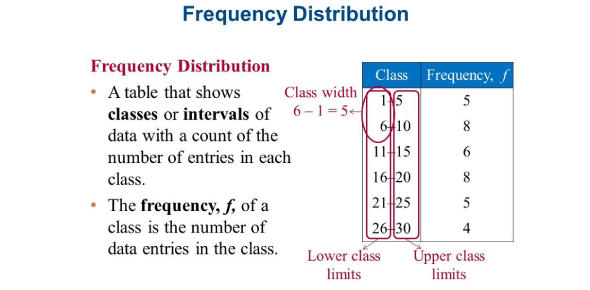# Frequency Distribution And Data Quiz

11 Questions | Attempts: 8833SettingsTake our frequency distribution and data quiz today to test yourself and learn more with the informative questions and answers. In a given sample there are some things that are the same in most of the variables within it. The number of instances in which a variable takes each of its possible values can be described by the frequency distribution. Take the test below and see how much you understand the frequency distribution shapes. All the best and keep on revising!

• 1.
Why is it sometimes easier to use a frequency table to interpret data than to examine the distribution of raw scores?
• A.

A frequency table transforms the raw scores by showing the means.

• B.

Frequency tables display patterns, organizing the data by how often each score occurs.

• C.

Raw scores are not based on the sample.

• D.

Raw scores do not represent the data.

• 2.
In comparing frequency tables with grouped frequency tables for the same set of data, which of the following would be true?
• A.

Depending on the width of class intervals it is possible that some scores may not be counted in a grouped frequency table.

• B.

If the data set is large the grouped frequency table would be easier to decipher.

• C.

Because of overlapping class intervals, the total frequency for a grouped frequency table would exceed that of a frequency table.

• D.

It is generally harder to spot patterns in the data when using a grouped frequency table.

• 3.
A bar graph is usually used with __________ data while a histogram is used with _________ data.
• A.

Interval; nominal

• B.

Ratio; scale

• C.

Nominal; scale

• D.

Nominal; ordinal

• 4.
A normal distribution is:
• A.

Symmetric.

• B.

Bell-shaped, symmetric, and unimodal.

• C.

Unimodal.

• D.

Bell-shaped.

• 5.
A distribution containing a few extremely small values is likely to create a distribution that is:
• A.

Negatively skewed.

• B.

Normal.

• C.

Positively skewed.

• D.

Non-representative.

• 6.
A frequency distribution that is bell-shaped, symmetric, and unimodal is called a:
• A.

Skewed distribution.

• B.

Sampling distribution.

• C.

Probability distribution.

• D.

Normal distribution.

• 7.
Imagine a researcher found that 65% of bystanders helped a female who dropped her packages but only 32% helped a male that dropped his packages. What type of graph would be appropriate to display this data?
• A.

Bar graph

• B.

Frequency polygon

• C.

Not enough information is available to answer the question

• D.

Histogram

• 8.
Imagine that you calculated Body Mass Index (BMI) for a group of college students and created the grouped frequency table below: BMI Frequency 40-44.99 1 35-39.99 4 30-34.99 6 25-29.99 10 20-24.99 20 15-19.99 3 If you decide to depict this data with a histogram what would be the midpoint for the highest interval?
• A.

42.5

• B.

40

• C.

42.49

• D.

44.99

• 9.
Professor Arenas recently administered a statistics exam and was surprised to see that the distribution of scores was negatively skewed. What would a negatively skewed distribution indicate for this example?
• A.

That most students did well on the exam but a few did very poorly.

• B.

That students were at the extreme; some did very well and others did very poorly.

• C.

That most students did poorly on the exam but a few did very well.

• D.

That there was very little variation in his class; everyone scored about the same.

• 10.
A positively-skewed distribution is more likely to occur whenever we have:
• A.

Floor effects.

• B.

A large sample size.

• C.

A wide variety of participants.

• D.

Ceiling effects.

• 11.
If students in a college math class are given an exam intended for middle school math students it would probably result in:
• A.

A positively skewed distribution.

• B.

A ceiling effect.

• C.

A normal distribution.

• D.

A floor effect.

## Related TopicsBack to top
×

Wait!
Here's an interesting quiz for you.